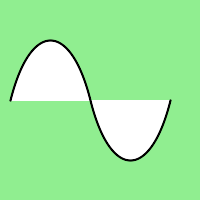Related Articles

# Wand path_curve() function in Python

• Last Updated : 20 Jun, 2021

path_curve() is a function specially introduced for paths. path_curve() draws a cubic bezier curve from the destination point of the Image to a particular point (x, y) with the help of control points.

Syntax :

`wand.drawing.path_curve(to, controls, smooth, relative)`

Parameters:

Example #1:

## Python3

 `from` `wand.image ``import` `Image``from` `wand.drawing ``import` `Drawing``from` `wand.color ``import` `Color` `with Drawing() as draw:``    ``draw.stroke_width ``=` `2``    ``draw.stroke_color ``=` `Color(``'black'``)``    ``draw.fill_color ``=` `Color(``'white'``)``    ``draw.path_start()``    ``# Start middle-left``    ``draw.path_move(to ``=``(``10``, ``100``))``    ``# Curve across top-left to center``    ``draw.path_curve(to ``=``(``80``, ``0``),``                    ``controls ``=``[(``20``, ``-``80``), (``60``, ``-``80``)],``                    ``relative ``=` `True``)``    ``# Continue curve across bottom-right``    ``draw.path_curve(to ``=``(``80``, ``0``),``                    ``controls ``=``(``60``, ``80``),``                    ``smooth ``=` `True``,``                    ``relative ``=` `True``)``    ``draw.path_finish()``    ``with Image(width ``=` `200``, height ``=` `200``, background ``=` `Color(``'lightgreen'``)) as image:``        ``draw(image)``        ``image.save(filename ``=``"pathcurve.png"``)`

Output :Attention geek! Strengthen your foundations with the Python Programming Foundation Course and learn the basics.

To begin with, your interview preparations Enhance your Data Structures concepts with the Python DS Course. And to begin with your Machine Learning Journey, join the Machine Learning – Basic Level Course

My Personal Notes arrow_drop_up